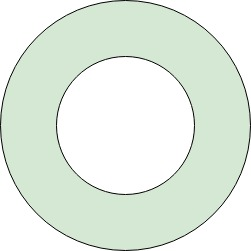# Program to calculate the area between two Concentric Circles

Given two concentric circles with radius X and Y where (X > Y). Find the area between them.

You are required to find the area of the green region as shown in the following image:Examples:

```Input : X = 2, Y = 1
Output : 9.42478

Input : X = 4, Y = 2
Output : 37.6991
```

## Recommended: Please try your approach on {IDE} first, before moving on to the solution.

Approach:

The area between the two given concentric circles can be calculated by subtracting the area of the inner circle from the area of the outer circle. Since X>Y. X is the radius of the outer circle.

Therefore, area between the two given concentric circles will be:

```π*X2 - π*Y2
```

Below is the implementation of the above approach:

## C++

 `// C++ program to find area between the ` `// two given concentric circles ` ` `  `#include ` `using` `namespace` `std; ` ` `  `// Function to find area between the ` `// two given concentric circles ` `double` `calculateArea(``int` `x, ``int` `y) ` `{ ` `    ``// Declare value of pi ` `    ``double` `pi = 3.1415926536; ` ` `  `    ``// Calculate area of outer circle ` `    ``double` `arx = pi * x * x; ` ` `  `    ``// Calculate area of inner circle ` `    ``double` `ary = pi * y * y; ` ` `  `    ``// Difference in areas ` `    ``return` `arx - ary; ` `} ` ` `  `// Driver Program ` `int` `main() ` `{ ` `    ``int` `x = 2; ` `    ``int` `y = 1; ` ` `  `    ``cout << calculateArea(x, y); ` ` `  `    ``return` `0; ` `} `

## Java

 `// Java program to find area between the ` `// two given concentric circles ` `import` `java.io.*; ` ` `  `class` `GFG  ` `{ ` `     `  `// Function to find area between the ` `// two given concentric circles ` `static` `double` `calculateArea(``int` `x, ``int` `y) ` `{ ` `    ``// Declare value of pi ` `    ``double` `pi = ``3.1415926536``; ` ` `  `    ``// Calculate area of outer circle ` `    ``double` `arx = pi * x * x; ` ` `  `    ``// Calculate area of inner circle ` `    ``double` `ary = pi * y * y; ` ` `  `    ``// Difference in areas ` `    ``return` `arx - ary; ` `} ` ` `  `// Driver code ` `public` `static` `void` `main (String[] args)  ` `{ ` `    ``int` `x = ``2``; ` `    ``int` `y = ``1``; ` `    ``System.out.println (calculateArea(x, y)); ` `} ` `} ` ` `  `// This code is contributed by jit_t. `

## Python 3

 `# Python3 program to find area between   ` `# the two given concentric circles  ` ` `  `# Function to find area between the  ` `# two given concentric circles  ` `def` `calculateArea(x, y):  ` ` `  `    ``# Declare value of pi  ` `    ``pi ``=` `3.1415926536` ` `  `    ``# Calculate area of outer circle  ` `    ``arx ``=` `pi ``*` `x ``*` `x  ` ` `  `    ``# Calculate area of inner circle  ` `    ``ary ``=` `pi ``*` `y ``*` `y  ` ` `  `    ``# Difference in areas  ` `    ``return` `arx ``-` `ary  ` ` `  `# Driver Code ` `x ``=` `2` `y ``=` `1` ` `  `print``(calculateArea(x, y))  ` ` `  `# This code is contributed  ` `# by shashank_sharma `

## C#

 `// C# program to find area between the ` `// two given concentric circles ` `using` `System; ` ` `  `class` `GFG  ` `{ ` `     `  `// Function to find area between the ` `// two given concentric circles ` `static` `double` `calculateArea(``int` `x, ``int` `y) ` `{ ` `    ``// Declare value of pi ` `    ``double` `pi = 3.1415926536; ` ` `  `    ``// Calculate area of outer circle ` `    ``double` `arx = pi * x * x; ` ` `  `    ``// Calculate area of inner circle ` `    ``double` `ary = pi * y * y; ` ` `  `    ``// Difference in areas ` `    ``return` `arx - ary; ` `} ` ` `  `// Driver code ` `public` `static` `void` `Main ()  ` `{ ` `    ``int` `x = 2; ` `    ``int` `y = 1; ` `    ``Console.WriteLine(calculateArea(x, y)); ` `} ` `} ` ` `  `// This code is contributed by Code_Mech. `

## PHP

 ` `

Output:

```9.42478
```

Time Complexity: O(1)

Attention reader! Don’t stop learning now. Get hold of all the important DSA concepts with the DSA Self Paced Course at a student-friendly price and become industry ready.

My Personal Notes arrow_drop_upCheck out this Author's contributed articles.

If you like GeeksforGeeks and would like to contribute, you can also write an article using contribute.geeksforgeeks.org or mail your article to contribute@geeksforgeeks.org. See your article appearing on the GeeksforGeeks main page and help other Geeks.

Please Improve this article if you find anything incorrect by clicking on the "Improve Article" button below.

Article Tags :
Practice Tags :

Be the First to upvote.

Please write to us at contribute@geeksforgeeks.org to report any issue with the above content.